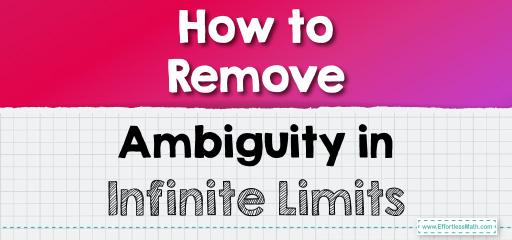# How to Remove Ambiguity in Infinite Limits

Let’s delve into the intricate process of resolving ambiguities inherent in infinite limits. The complexities of infinity in calculus often manifest in indeterminate forms that cannot be directly solved. Among these are the perplexing $$0×∞$$ and $$\frac{∞}{∞}$$ types. Here's a detailed guide to navigate through such ambiguities, often with the goal of transforming them into a $$\frac{0}{0}$$ indeterminate form, which is amenable to techniques such as L'Hôpital's Rule.## Step-by-step Guide to Remove Ambiguity in Infinite Limits

Here is a step-by-step guide to removing ambiguity in infinite limits:

### Step 1: Understanding the Ambiguity of Infinity

Firstly, grasp that the expressions involving infinity ($$∞$$) are not definite numbers but represent the idea of unbounded increase or decrease. Ambiguous forms like $$0×∞$$ and $$\frac{∞}{∞}$$ require a strategy that transforms them into a solvable structure, typically the $$\frac{0}{0}$$ indeterminate form.

### Step 2: Establishing General Principles for Infinity

Assume that $$lim_{x→c​}f(x)=±∞$$ and $$lim_{x→c​}g(x)=L$$, where $$L$$ is a real number and not zero. Here, we have the following conditions:

• For $$L>0$$, $$lim_{x→c}​[f(x)×g(x)]=±∞$$ and $$lim_{x→c}​[\frac{f(x)}{g(x)}]=±∞$$.
• For $$L<0$$, $$lim_{x→c}​[f(x)×g(x)]=∓∞$$ and $$lim_{x→c}​[\frac{f(x)}{g(x)}]=∓∞$$.
• Addition or subtraction with $$±∞$$ retains the infinity.

If both functions approach positive infinity, then the product and sum also tend to be positive infinity.

### Step 3: Resolving the $$0×∞$$ Indeterminate Form

When L is zero, we face the $$0×∞$$ ambiguity. To address this, we aim to rewrite the expression to create a $$\frac{0}{0}$$ form. Here’s how:

Transform $$lim_{x→c}​[f(x)×g(x)]$$ into $$lim_{x→c​}$$$$[\frac{g(x)}{(\frac{1}{f(x)}​)}]$$. Since $$\frac{real \ number}{∞}=0$$, this transformation can yield a $$\frac{0}{0}$$ scenario, which is more manageable.

### Step 4: Addressing the $$\frac{∞}{∞}$$ Indeterminate Form

When confronting the $$\frac{∞}{∞}$$ form, we apply a similar tactic by inverting both the numerator and the denominator, transforming $$lim_{x→c}​[f(x)×g(x)]$$ into $$lim_{x→c​}$$$$[\frac{\frac{1}{g(x)}}{(\frac{1}{f(x)})}]$$, thus aiming for a $$\frac{0}{0}$$ condition.

### Step 5: Simplification to Escape Infinity Ambiguity

Simplify the expressions where possible:

• Factorize polynomials to cancel common terms.
• Divide each term in a rational function by the highest power of $$x$$ in the denominator to normalize the limit expression.

### Step 6: Applying L’Hôpital’s Rule

Once a $$\frac{0}{0}$$ indeterminate form is achieved through the steps above, L’Hôpital’s Rule can be applied. This involves taking the derivative of the numerator and the derivative of the denominator and then re-evaluating the limit.

### Step 7: Continuous Evaluation and Transformation

The process might need to be iterated: simplification, applying L’Hôpital’s Rule, or further transformation to expose a $$\frac{0}{0}$$ form may need to be performed multiple times.

## Final Word:

By methodically transforming indeterminate forms involving infinity into the more tractable $$\frac{0}{0}$$ form and employing strategic calculus tools, the veil of ambiguity is lifted, allowing for the precise determination of limits at infinity. This mastery over the ethereal concept of infinity is a testament to the logical structure and elegance of mathematical principles, even when they stretch into the boundless.

### What people say about "How to Remove Ambiguity in Infinite Limits - Effortless Math: We Help Students Learn to LOVE Mathematics"?

No one replied yet.

X
30% OFF

Limited time only!

Save Over 30%

SAVE $5 It was$16.99 now it is \$11.99